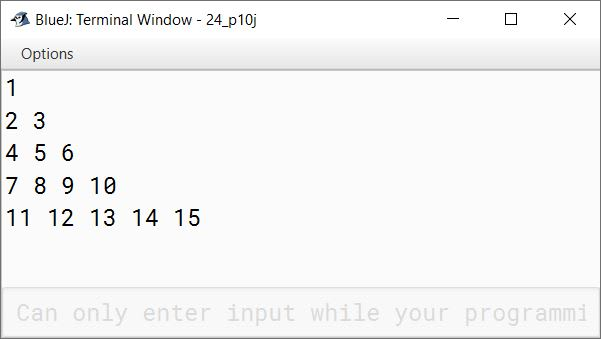# Nested for Loops

## Fill in the blanks

#### Question 1

A loop within another loop is called nested loops

#### Question 2

You can use break statement to terminate a loop block.

#### Question 3

Label is a tag that decides which of part the loop is to be used.

#### Question 4

Repetition of inner loop takes place before outer loop

#### Question 5

Continue statement will repeat a loop for next iteration after ignoring some statements of the loop.

## Write whether the following statements are True/False

#### Question 1

Loop is a repetitive structure.
True

#### Question 2

Nesting of loops means restricting the use of inner loop.
False

#### Question 3

When break statement is applied, it terminates the program completely.
False

#### Question 4

When outer loop completes its iterations, the inner loop starts. False

#### Question 5

Labelled continue statement allows the next iteration of the loop from any place of looping structure.
False

## Differentiate between the following

#### Question 1

Nested if and nested loop
Nested if is used to do conditional checks at multiple levels whereas nested loops are used to execute one iterative set of statements inside another iterative set.

#### Question 2

Break and continue

1. break statement is used to unconditionally jump out of the loop whereas continue statement is used to unconditionally jump to the next iteration of the loop, skipping the remaining statements of the current iteration.
2. break statement is used in switch-case and loops whereas continue statement is only used in loops.

#### Question 3

Labelled break and Unlabelled break
Labelled break can be used to exit out of a deeply nested set of loops whereas Unlabelled break only exits from the loop within which it is enclosed.

#### Question 1

What do you mean by a nested loop?
When a loop is contained inside another loop it is termed as nested loops

#### Question 2

In what situation you need a nested loop?
When the repetition of two tasks depend on each other in such a way that for every repetition of first task, the second tasks needs to be repeated a number of times, then we use nested loops.

#### Question 3

How will you terminate outer loop from the block of the inner loop?
By using Labelled break statement.

#### Question 4

What do you mean by labelled break statement? Give an example.
Labelled break statement transfers program control out of the code block whose label is specified as its target. The target code block must enclose the break statement but it does not need to be the immediately enclosing block.
In the below code snippet:

```first: for (int j = 1; j <= 5; j++) {
for (int k = 1; k <= j; k++) {
if (k > 4)
break first;
System.out.print(k);
}
System.out.println();
}
System.out.println("Outside code block labelled first");```

the labelled break statement `break first;` will transfer the program control outside the outer for loop to the statement `System.out.println("Outside code block labelled first");`

#### Question 5

Write down the syntax of the nested loop.
Below is the syntax of nested loop:

```
for (<initial value>; <test condition>; <update value>) {
for (<initial value>; <test condition>; <update value>) {
executable statement(s)
}
}
```

## Give the output of the following snippets based on nested loops

#### Question 1

```int i,j;
for (i=0; i<4; i++)
{
for (j=i; j>=0; j--)
System.out.print(j);
System.out.println();
}```
```0
10
210
3210
```
##### Explanation

For each iteration of outer for loop, inner for loop will iterate from i to 0 printing the above pattern.

#### Question 2

```int y,p;
for (int x=1; x<=3; x++)
{
for (y=1; y<=2; y++)
{
p = x * y;
System.out.print(p);
}
System.out.println( );
}```
```12
24
36
```
##### Explanation
xypRemarks
1111st iteration of outer for loop
22
2122nd iteration of outer for loop
24
3133rd iteration of outer for loop
26

#### Question 3

```int a,b;
for (a=1; a<=2; a++)
{
for (b= (64+a); b<=70; b++)
System.out.print((char) b);
System.out.println( );
}```
##### Output
```ABCDEF
BCDEF
```
##### Explanation

In the first iteration of outer for loop, the inner for loop will print characters with ASCII codes from 65 to 70 i.e. letters from A to F.
In the second iteration of outer for loop, the inner for loop will print characters with ASCII codes from 66 to 70 i.e. letters from B to F.

#### Question 4

```int x,y;
for(x=1; x<=5; x++)
{
for(y=1; y<x; y++)
{
if(x == 4)
break;
System.out.print(y);
}
System.out.println( );
}```
```
1
12

1234
```
##### Explanation

1st iteration of outer for
x = 1
Inner for loop doesn't execute as y = 1 so the condition y<x is false
Just a newline is printed to the console due to `System.out.println( );`

2nd iteration of outer for
x = 2
Inner for loop executes once printing 1 to the console

3rd iteration of outer for
x = 3
Inner for loop executes twice printing 12 to the console

4th iteration of outer for
x = 4
`if(x == 4)` becomes true inside inner for loop. `break` is executed, just a newline is printed to the console.

5th iteration of outer for
x = 5
Inner for loop executes 4 times printing 1234 to the console

#### Question 5

```int i,j;
first:
for (i=10; i>=5; i--)
{
for (j= 5; j<=i; j++)
{
if (i*j <40)
continue first;
System.out.print(j);
}
System.out.println( );
}```
##### Output
```5678910
56789
5678

```
##### Explanation

For the first 3 iterations of outer loop i * j >= 40. After that as the condition of `if (i*j <40)` becomes true, in each iteration of inner for, continue statement transfers the program control to the next iteration of outer for loop.

## Solutions to Unsolved Java Programs

#### Question 1

Write a program to display the Mathematical Table from 5 to 10 for 10 iterations in the given format:
Sample Output: Table of 5
5*1 = 5
5*2 =10
--------
--------
5*10 = 50

```public class KboatTables
{
public static void main(String args[]) {
for (int i = 5; i <= 10; i++) {
System.out.println("Table of " + i);
for (int j = 1; j <= 10; j++) {
System.out.println(i + "*" + j + " = " + (i*j));
}
}
}
}```
##### Output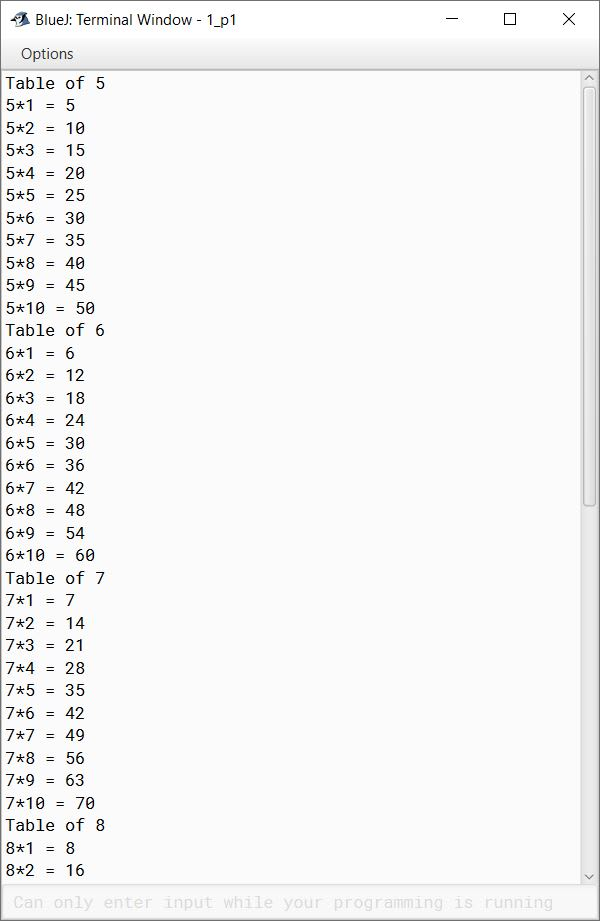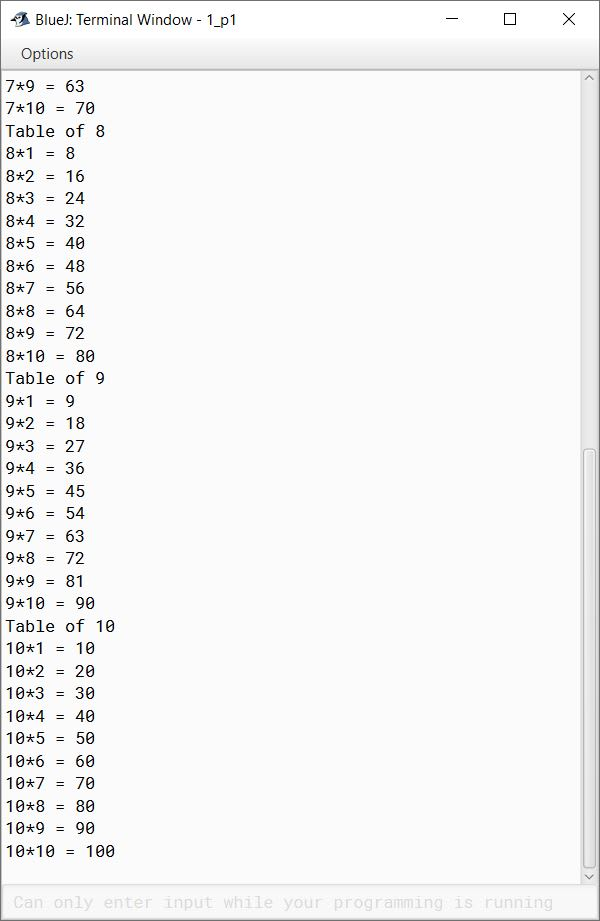#### Question 2

Write a program to accept any 20 numbers and display only those numbers which are prime.
Hint: A number is said to be prime if it is only divisible by 1 and the number itself.

```import java.util.Scanner;

public class KboatPrimeCheck
{
public static void main(String args[]) {
Scanner in = new Scanner(System.in);
System.out.println("Enter 20 numbers");
for (int i = 1; i <= 20; i++) {
int n = in.nextInt();
boolean isPrime = true;
for (int j = 2; j <= n / 2; j++) {
if (n % j == 0) {
isPrime = false;
break;
}
}
if (isPrime)
System.out.println(n + " is a Prime Number");
}
}
}```
##### Output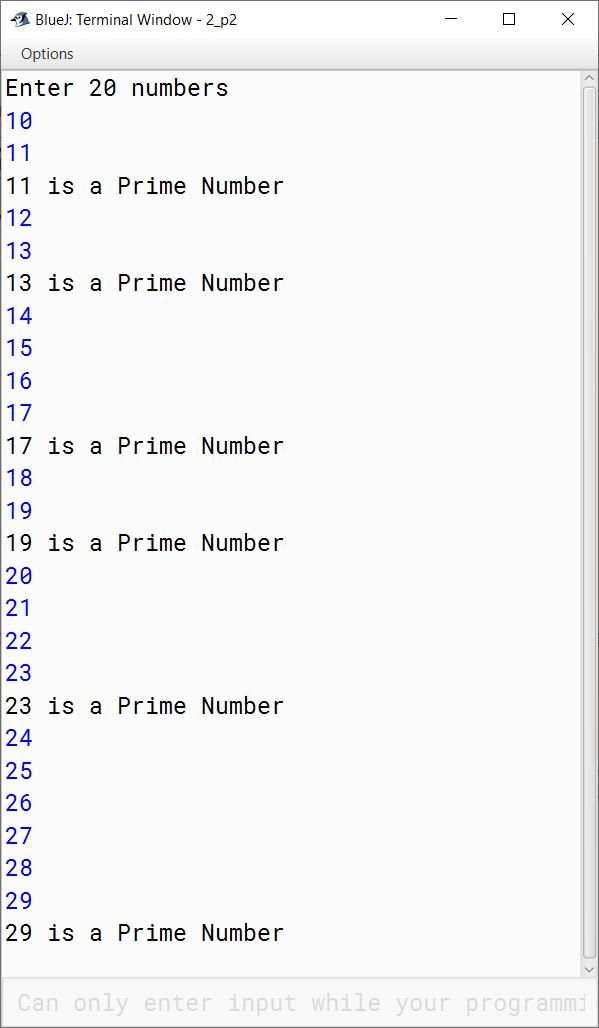#### Question 3

Write a program to compute and display the sum of the following series:
S = (1 + 2) / (1 * 2) + (1 + 2 + 3) / (1 * 2 * 3) + -------- + (1 + 2 + 3 + ----- + n ) / (1 * 2 * 3 * ----- * n)

```import java.util.Scanner;

public class KboatSeries
{
public static void main(String args[]) {
Scanner in = new Scanner(System.in);
System.out.print("Enter n: ");
int n = in.nextInt();
double sum = 0.0;
for (int i = 2; i <= n; i++) {
double num = 0.0, den = 1.0;
for (int j = 1; j <= i; j++) {
num += j;
den *= j;
}
sum = sum + (num / den);
}
System.out.println("Sum=" + sum);
}
}```
##### Output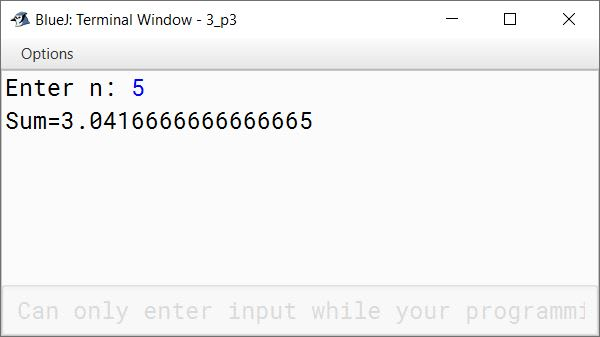#### Question 4

Write two separate programs to generate the following patterns using iteration (loop) statements:

(a)

*
*  #
*  #  *
*  #  *  #
*  #  *  #  *

```public class KboatPattern
{
public static void main(String args[]) {
for (int i = 1; i <=5; i++) {
for (int j = 1; j <= i; j++) {
if (j % 2 == 0)
System.out.print("# ");
else
System.out.print("* ");
}
System.out.println();
}
}
}```
##### Output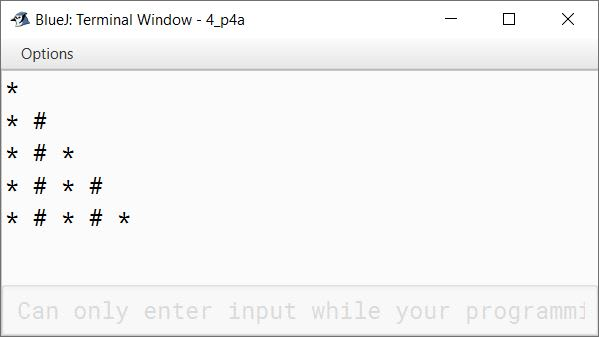(b)

5 4 3 2 1
5 4 3 2
5 4 3
5 4
5

```public class KboatPattern
{
public static void main(String args[]) {
for (int i = 1; i <=5; i++) {
for (int j = 5; j >= i; j--) {
System.out.print(j + " ");
}
System.out.println();
}
}
}```
##### Output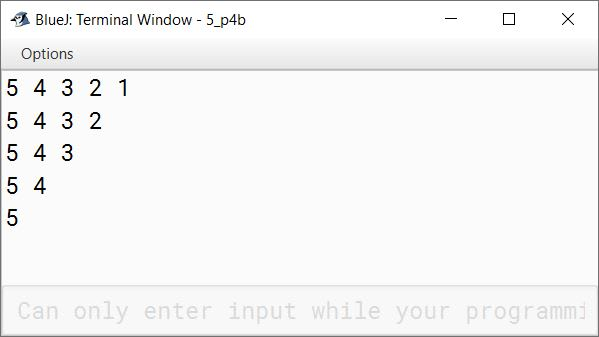#### Question 5

Write a program to calculate and display the factorials of all the numbers between 'm' and 'n' (where m<n, m>0, n>0).
[Hint: factorial of 5 means: 5!=5*4*3*2*1]

```import java.util.Scanner;

public class KboatFactRange
{
public static void main(String args[]) {

Scanner in = new Scanner(System.in);
System.out.print("Enter m: ");
int m = in.nextInt();
System.out.print("Enter n: ");
int n = in.nextInt();

if (m < n && m > 0 && n > 0) {
for (int i = m; i <= n; i++) {
long fact = 1;
for (int j = 1; j <= i; j++)
fact *= j;
System.out.println("Factorial of " + i + " = " + fact);
}
}
else {
System.out.println("Invalid Input");
}

}
}```
##### Output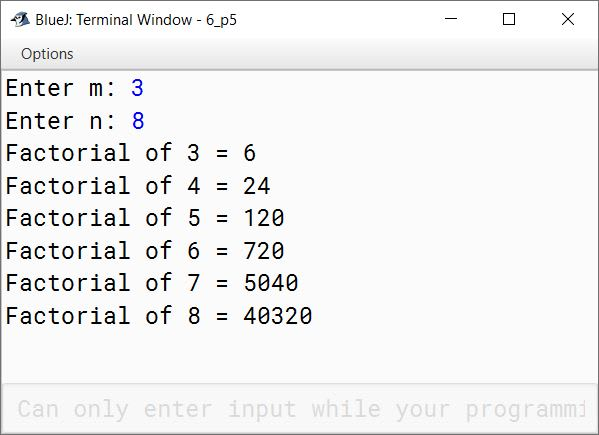#### Question 6

Write a menu driven program to display all prime and non-prime numbers from 1 to 100.
Enter 1: to display all prime numbers
Enter 2: to display all non-prime numbers
Hint: A number is said to be prime if it is only divisible by 1 and the number itself.

```import java.util.Scanner;

{
public static void main(String args[]) {
Scanner in = new Scanner(System.in);
System.out.println("Enter 1: to display all prime numbers");
System.out.println("Enter 2: to display all non-prime numbers");
int choice = in.nextInt();

switch (choice) {
case 1:
for (int i = 2; i <= 100; i++) {
boolean isPrime = true;
for (int j = 2; j <= i / 2; j++) {
if (i % j == 0) {
isPrime = false;
break;
}
}
if (isPrime)
System.out.println(i);
}
break;

case 2:
System.out.println(1);
for (int i = 2; i <= 100; i++) {
boolean isPrime = true;
for (int j = 2; j <= i / 2; j++) {
if (i % j == 0) {
isPrime = false;
break;
}
}
if (!isPrime)
System.out.println(i);
}
break;

default:
System.out.println("Incorrect Choice");
break;
}
}
}```
##### Output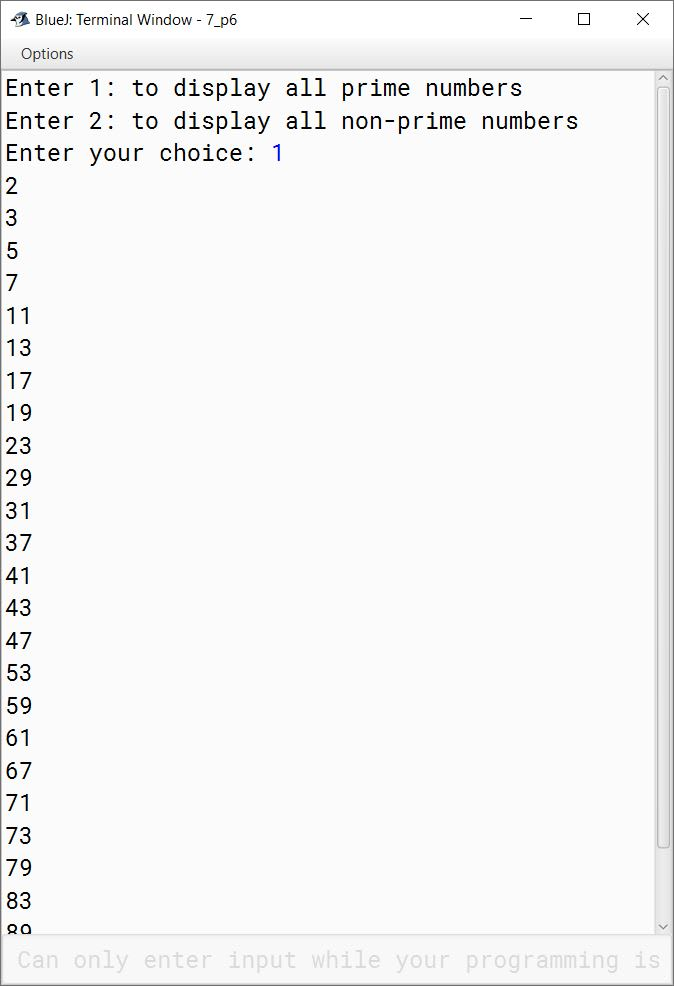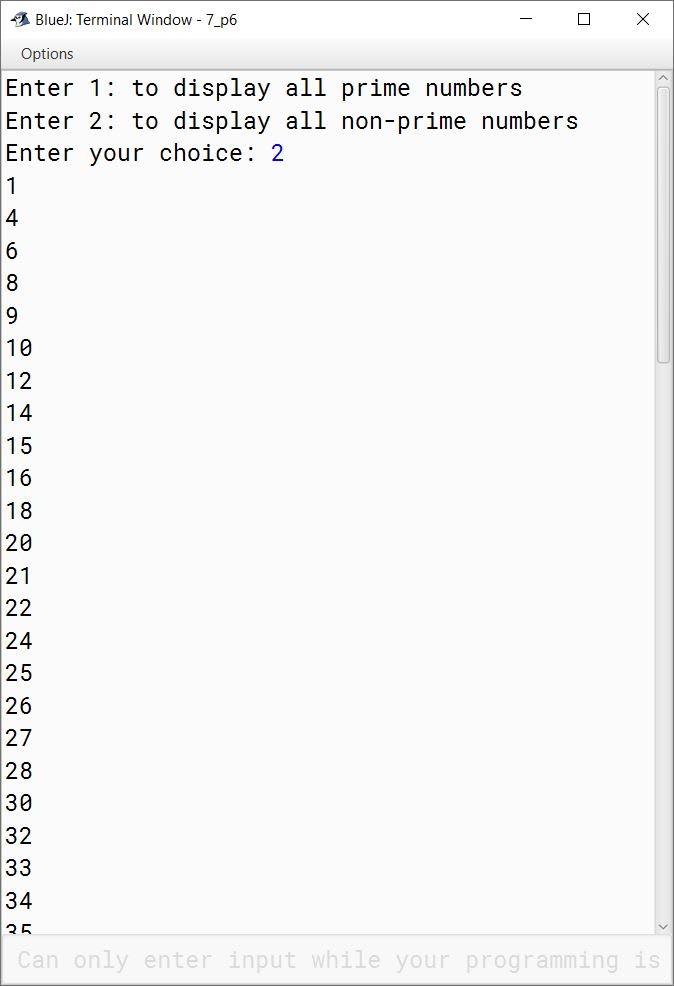#### Question 7

In an entrance examination, students have answered English, Maths and Science papers. Write a program to calculate and display average marks obtained by all the students. Take number of students appeared and marks obtained in all three subjects by every student along with the name as inputs.

```import java.util.Scanner;

public class KboatStudentMarks
{
public static void main(String args[]) {
Scanner in = new Scanner(System.in);
System.out.print("Enter number of students: ");
int studentCount = in.nextInt();
double totalMarks = 0.0;

for (int i = 1; i <= studentCount; i++) {
System.out.println("Enter details of student " + i);
System.out.print("Name: ");
in.nextLine();
String name = in.nextLine();
System.out.print("Marks in English: ");
int engMarks = in.nextInt();
System.out.print("Marks in Science: ");
int sciMarks = in.nextInt();
System.out.print("Marks in Maths: ");
int mathsMarks = in.nextInt();
double avgMarks = (engMarks + sciMarks + mathsMarks) / 3.0;
totalMarks += avgMarks;
System.out.println("Average marks of " + name + " = " + avgMarks);
}

double classAvg = totalMarks / studentCount;
System.out.println("Class Average = " + classAvg);
}
}```
##### Output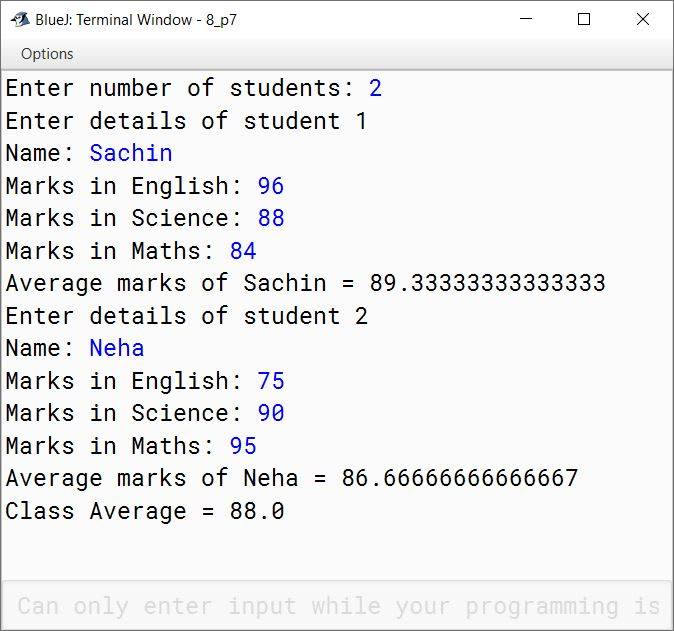#### Question 8

Write a program to input a number and perform the following tasks:
(a) to check whether it is a prime number or not
(b) to reverse the number
If the number as well as the reverse is also 'Prime' then display 'Twisted Prime' otherwise 'Not a twisted Prime'.
Sample Input: 167
Sample Output: 167 and 761 both are prime.
It is a 'Twisted Prime'.

```import java.util.Scanner;

public class KboatTwistedPrime
{
public static void main(String args[]) {
Scanner in = new Scanner(System.in);
System.out.print("Enter number: ");
int num = in.nextInt();

if (num == 1) {
System.out.println(num + " is not a twisted prime number");
}
else {
boolean isPrime = true;
for (int i = 2; i <= num / 2; i++) {
if (num % i == 0) {
isPrime = false;
break;
}
}

if (isPrime) {

int t = num;
int revNum = 0;

while (t != 0) {
int digit = t % 10;
t /= 10;
revNum = revNum * 10 + digit;
}

for (int i = 2; i <= revNum / 2; i++) {
if (revNum % i == 0) {
isPrime = false;
break;
}
}
}

if (isPrime)
System.out.println(num + " is a twisted prime number");
else
System.out.println(num + " is not a twisted prime number");
}

}
}```
##### Output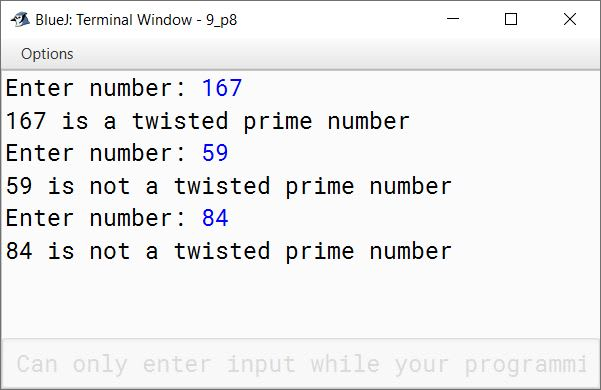#### Question 9

Write programs to find the sum of the given series:

(a) 1 + (1/2!) + (1/3!) + (1/4!) + .......... + (1/n!)

```import java.util.Scanner;

public class KboatSeriesSum
{
public static void main(String args[]) {
Scanner in = new Scanner(System.in);
System.out.print("Enter n: ");
int n = in.nextInt();
double sum = 0.0;
for (int i = 1; i <= n; i++) {
long f = 1;
for (int j = 1; j <= i; j++) {
f *= j;
}
sum += (1.0 / f);
}
System.out.println("Sum=" + sum);
}
}```
##### Output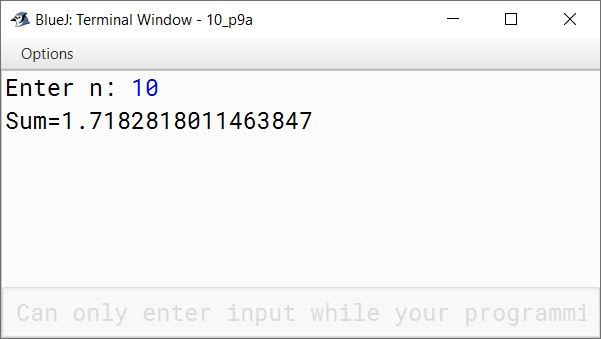(b) 1 + (1+2) + (1+2+3) + .......... + (1+2+3+ ...... + n)

```import java.util.Scanner;

public class KboatSeriesSum
{
public static void main(String args[]) {
Scanner in = new Scanner(System.in);
System.out.print("Enter n: ");
int n = in.nextInt();
long sum = 0;
for (int i = 1; i <= n; i++) {
for (int j = 1; j <= i; j++) {
sum += j;
}
}
System.out.println("Sum=" + sum);
}
}```
##### Output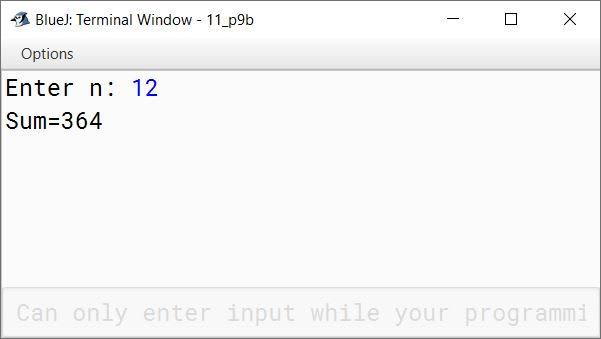(c) 1 + (1*2) + (1*2*3) + .......... + (1*2*3* ...... * n)

```import java.util.Scanner;

public class KboatSeriesSum
{
public static void main(String args[]) {
Scanner in = new Scanner(System.in);
System.out.print("Enter n: ");
int n = in.nextInt();
long sum = 0;
for (int i = 1; i <= n; i++) {
int p = 1;
for (int j = 1; j <= i; j++) {
p *= j;
}
sum += p;
}
System.out.println("Sum=" + sum);
}
}```
##### Output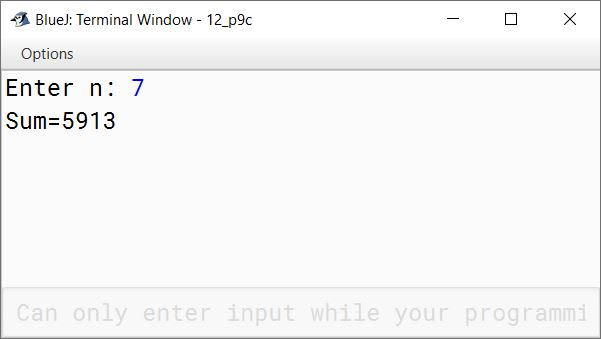(d) 1 + 1 / (1+2) + 1 / (1+2+3) + .......... + 1 / (1+2+3+.....+n)

```import java.util.Scanner;

public class KboatSeriesSum
{
public static void main(String args[]) {
Scanner in = new Scanner(System.in);
System.out.print("Enter n: ");
int n = in.nextInt();
double sum = 0.0;
for (int i = 1; i <= n; i++) {
long term = 0;
for (int j = 1; j <= i; j++) {
term += j;
}
sum += (1.0 / term);
}
System.out.println("Sum=" + sum);
}
}```
##### Output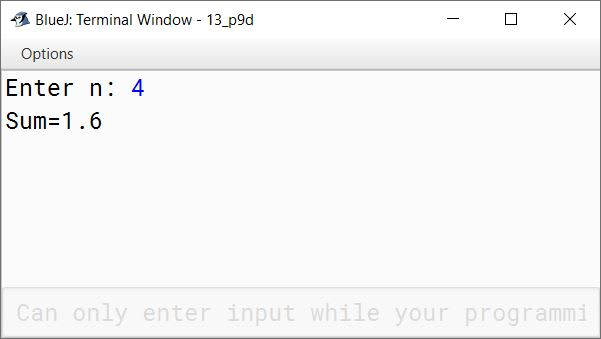(e) (1/2) + (1/3) + (1/5) + (1/7) + (1/11) + .......... + (1/29)

```public class KboatSeriesSum
{
public static void main(String args[]) {
double sum = 0.0;
for (int i = 2; i < 30; i++) {
boolean isPrime = true;
for (int j = 2; j <= i / 2; j++) {
if (i % j == 0) {
isPrime = false;
break;
}
}
if (isPrime)
sum += 1.0 / i;
}
System.out.println("Sum=" + sum);
}
}```
##### Output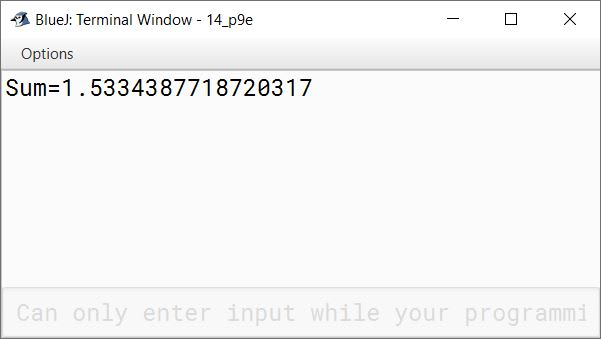#### Question 10

Write the programs in Java to display the following patterns:

(a)

1
2 1
3 2 1
4 3 2 1
5 4 3 2 1

```public class KboatPattern
{
public static void main(String args[]) {
for (int i = 1; i <= 5; i++) {
for (int j = i; j >= 1; j--) {
System.out.print(j + " ");
}
System.out.println();
}
}
}```
##### Output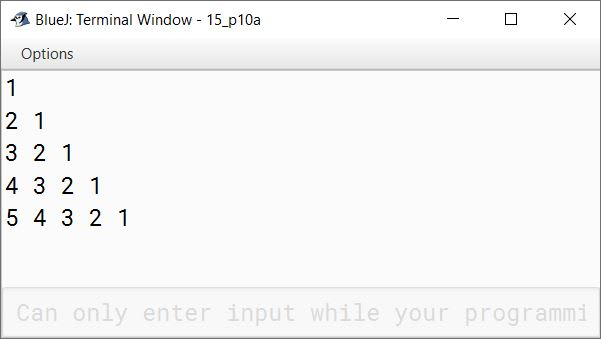(b)

1 2 3 4 5
1 2 3 4
1 2 3
1 2
1

```public class KboatPattern
{
public static void main(String args[]) {
for (int i = 5; i >= 1; i--) {
for (int j = 1; j <= i; j++) {
System.out.print(j + " ");
}
System.out.println();
}
}
}```
##### Output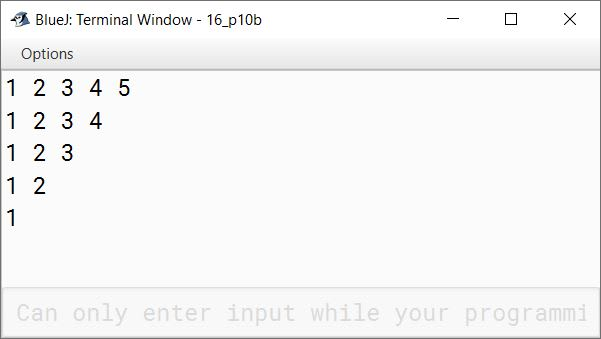(c)

5 4 3 2 1
5 4 3 2
5 4 3
5 4
5

```public class KboatPattern
{
public static void main(String args[]) {
for (int i = 1; i <= 5; i++) {
for (int j = 5; j >= i; j--) {
System.out.print(j + " ");
}
System.out.println();
}
}
}```
##### Output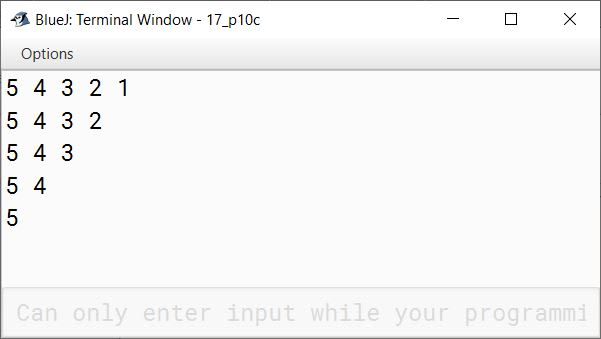(d)

1 3 5 7 9
1 3 5 7
1 3 5
1 3
1

```public class KboatPattern
{
public static void main(String args[]) {
for (int i = 9; i >= 1; i -= 2) {
for (int j = 1; j <= i; j += 2) {
System.out.print(j + " ");
}
System.out.println();
}
}
}```
##### Output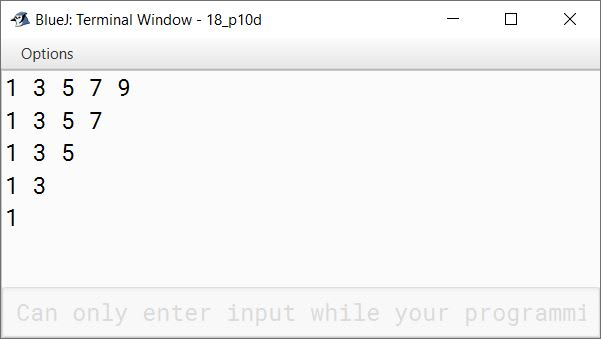(e)

5
5 4
5 4 3
5 4 3 2
5 4 3 2 1

```public class KboatPattern
{
public static void main(String args[]) {
for (int i = 5; i >= 1; i--) {
for (int j = 5; j >= i; j--) {
System.out.print(j + " ");
}
System.out.println();
}
}
}```
##### Output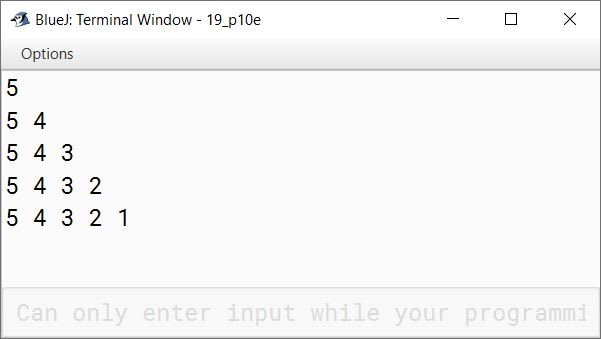(f)

1 2 3 4 5
2 3 4 5
3 4 5
4 5
5

```public class KboatPattern
{
public static void main(String args[]) {
for (int i = 1; i <= 5; i++) {
for (int j = i; j <= 5; j++) {
System.out.print(j + " ");
}
System.out.println();
}
}
}```
##### Output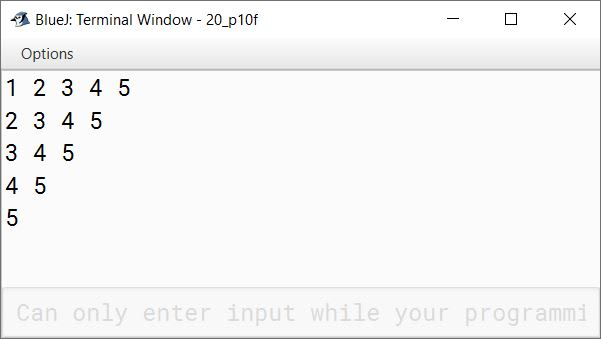(g)

9 9 9 9 9
7 7 7 7 7
5 5 5 5 5
3 3 3 3 3
1 1 1 1 1

```public class KboatPattern
{
public static void main(String args[]) {
for (int i = 9; i >= 1; i -= 2) {
for (int j = 1; j <= 5; j++) {
System.out.print(i + " ");
}
System.out.println();
}
}
}```
##### Output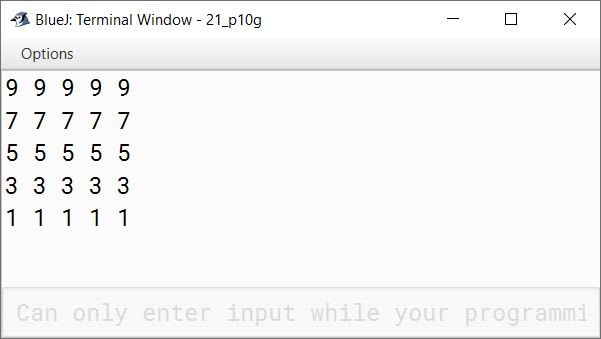(h)

9
7 9
5 7 9
3 5 7 9
1 3 5 7 9

```public class KboatPattern
{
public static void main(String args[]) {
for (int i = 9; i >= 1; i -= 2) {
for (int j = i; j <= 9; j += 2) {
System.out.print(j + " ");
}
System.out.println();
}
}
}```
##### Output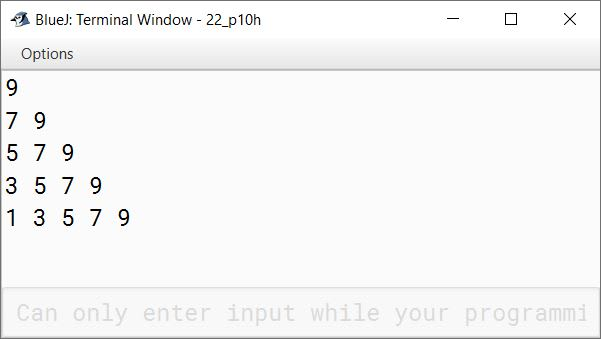(i)

9
9 7
9 7 5
9 7 5 3
9 7 5 3 1

```public class KboatPattern
{
public static void main(String args[]) {
for (int i = 9; i >= 1; i -= 2) {
for (int j = 9; j >= i; j -= 2) {
System.out.print(j + " ");
}
System.out.println();
}
}
}```
##### Output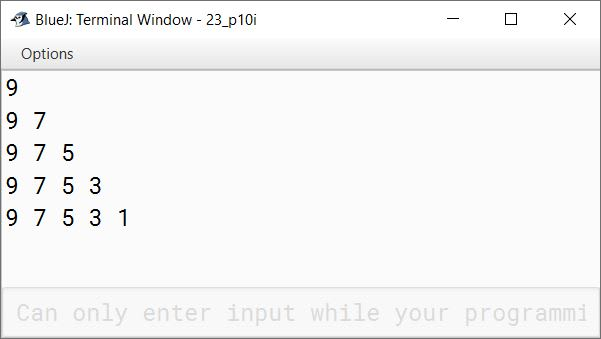(j)

1
2 3
4 5 6
7 8 9 10
11 12 13 14 15

```public class KboatPattern
{
public static void main(String args[]) {
int term = 1;
for (int i = 1; i <= 5; i++) {
for (int j = 1; j <= i; j++) {
System.out.print(term++ + " ");
}
System.out.println();
}
}
}```
##### Output